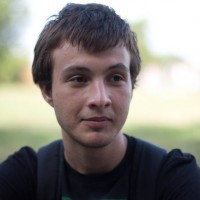Personal Websites

# Sergey Matveev

#### Professor: Ivan Oseledets

Specialist’s degree in Mathematics and System programming, Lomonosov Moscow State University (2015)
Candidate of Science in Physics and Mathematics, defended in Marchuck Institute of Numerical Mathematics, RAS (2018)
Post-graduate diploma of Lomonosov Moscow State University (2018)

Scopus Author ID: 56421723800

Sergey received his Specialist’s degree in Mathematics and System Programming from Lomonosov Moscow State University. As a research student he worked in Marchuk Institute of Numerical Mathematics RAS on elaboration and analysis of new fast numerical methods solving multidimensional Smoluchowski coagulation equation under guidance of prof. Eugene Tyrtyshnikov. In addition, he participated in elaboration of novel semiclassical Monte-Carlo methods modelling the non-adiabatic molecular dynamics at Los Alamos National Laboratory under guidance of Dmitry Mozyrsky in 2014, 2015 and 2016.

In 2015-2016 Sergey was a junior research scientist at CEE CREI working on elaboration of efficient computational tools for the molecular modelling.  In addition to research and programming duties Sergey has also helped to establish new computing cluster for CEE and optimize use of the existing computing facilities.

Since 2017 Sergey works as a member of group of Prof. Ivan Oseledets on research project devoted to monitoring of soil fertility factors and the analysis of the transformation of soil organic matter. During vacation time from Skoltech Sergey was 6-week visitor of CEES (Univ. of Oslo) and participated in joint Russian-Norway project devoted to mathematical modelling of Atlantic cod population dynamics.  In 2018 he defended Candidate of Science thesis (PhD equivalent) in Marchuck Institute of Numerical Mathematics devoted to elaboration and justification of the fast numerical methods for Smoluchowski-typed equations.

• Numerical linear algebra and tensor decompositions
• High-performance computing
• Parallel and distributed computing
• Computational physics
3. ###### R.R. Zagidullin, A. P. Smirnov, S. A. Matveev, E.E. Tyrtyshnikov, Supercomputer modelling of spatially-heterogeneous coagulation using MPI and CUDA, Springer CCIS, 2019
4. S. A. Matveev, A. P. Smirnov, E. E. Tyrtyshnikov, The effect of a monomer source on the oscillation period in an irreversible coagulation model, Computational Mathematics and Modeling, Vol 30, № 5, pp. 378-382, 2019
5. Mirvakhabova, M. Pukalchik, S. Matveev, P. Tregubova., I. Oseledets, Field heterogeneity detection based on the modified FastICA RGB-image processing, IOP Journal of Physics: Conference Series (Vol. 1117, No. 1, p. 012009).
6. S. A. Matveev, R. R. Zagidullin, A. P. Smirnov, E. E. Tyrtyshnikov, Parallel Numerical Algorithm for Solving Advection Equation for Coagulating Particles, Supercomputing Frontiers and Innovations vol.5 iss. 2, 2018
7. A. Somov, D. Shadrin, I. Fastovets, A. Nikitin, S. Matveev, I. Oseledets, O. Hrinchuk Pervasive Agriculture: IoT Enabled Greenhouse for Plant Growth Control , IEEE Pervasive Computing vol. 17, iss. 4, pp 65-75, 2018
8. N. V. Brilliantov, W. Otieno, S. A. Matveev, A. P. Smirnov, E. E. Tyrtyshnikov, PL Krapivsky, Steady oscillations in aggregation-fragmentation processes, Physical Review E, vol. 98, iss. 1, 2018
9. D. A. Stefonishin , S. A. Matveev , A. P. Smirnov, E. E. Tyrtyshnikov, An Efficient Finite-Difference Method for Solving Smoluchowski-Type Kinetic Equations of Aggregation with Three-Body Collisions, Numerical methods and programming, Vol 19, 261-269, 2018 (in Russian)
10. D. A. Stefonishin , S. A. Matveev , A. P. Smirnov, E. E. Tyrtyshnikov, Tensor decompositions for solving the equations of mathematical models of aggregation with multiple collisions of particles, Numerical methods and programming, Vol 19, 390-404 (in Russian)
11. S. A. Matveev, P. L. Krapivsky, A. P. Smirnov, E. E. Tyrtyshnikov, N. V. Brilliantov, Oscillations in aggregation-shattering processes, Physical Review Letters (2017), 119(26):260601
12. R. R. Zagidullin, A. P. Smirnov, S. A. Matveev, E. E. Tyrtyshnikov, An efficient numerical method for a mathematical model of a transport of coagulating particles, Moscow Univ. Comput. Math. Cybern. (2017) 41: 179.
13. SA Matveev, VI Stadnichuk, EE Tyrtyshnikov, AP Smirnov, NV Ampilogova, NV Brilliantov, Anderson acceleration method of finding steady-state particle size distribution for a wide class of aggregation-fragmentation models, Computer Physics Communications, vol. 224, 2018 (available online since Nov 2017)
14. Smirnov A. P., Matveev S. A., Zheltkov D. A., Tyrtyshnikov E. E., Fast and Accurate Finite-difference Method Solving Multicomponent Smoluchowski Coagulation Equation with Source and Sink Terms, Procedia Computer Science 80 (2016), 2141–2146
15. Matveev S. A., Zheltkov D. A., Tyrtyshnikov E. E., Smirnov A. P., “Tensor train versus Monte Carlo for the multicomponent Smoluchowski coagulation equation.”Journal of Computational Physics 316 (2016): 164-179
16. Matveev S. A., A Parallel Implementation of a Fast Method for Solving the Smoluchowski-Type Kinetic Equations of Aggregation and Fragmentation processes, Numerical methods and programming, Vol. 15, p. 360 – 368, 2015 (in Russian).
17. Matveev S. A., Smirnov A. P., Tyrtyshnikov E. E. “A fast numerical method for the Cauchy problem for the Smoluchowski equation.” Journal of Computational Physics 282 (2015): 23-32.
18. Matveev S.A., Tyrtyshnikov E.E., Smirnov A.P., Brilliantov N.V., A fast numerical method for solving the Smoluchowski-type kinetic equations of aggregation and fragmentation processes, Numerical methods and programming, Vol. 15, p 1-8, 2014 (in Russian).
• Mathematical modelling
• Fast numerical methods for Smouchowski-typed kinetic equations
• Spatially heterogeneous models of aggregation and fragmentation processes
• Low-rank matrix and tensor decompositions

2017 — Governmental stipend for successful PhD-students

2015 — Gold medal of Russian Academy of Sciences for the best student research work in mathematics

2015 — First prize in diploma competition of the faculty of Computational Mathematics and Cybernetics of Lomonosov Moscow State University